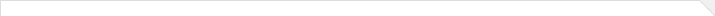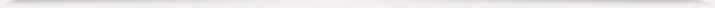E-mail：sjtang AT fudan.edu.cn1. Shanjian Tang,  Brockett's problem of classification of finite-dimensional estimation algebras for nonlinear filtering systems. SIAM J. Control Optim. 39 (2000), no. 3, 900–916.
2. Shanjian Tang, General linear quadratic optimal stochastic control problems with random coefficients: linear stochastic Hamilton systems and backward stochastic Riccati equations. SIAM J. Control Optim. 42 (2003), no. 1, 53–75.
3. Shanjian Tang, Semi-linear systems of backward stochastic partial differential equations in \$R^n\$ . Chinese Ann. Math. Ser. B 26 (2005), no. 3, 437–456.
4. Freddy Delbaen and Shanjian Tang, Harmonic analysis of stochastic equations and backward stochastic differential equations. Probab. Theory Related Fields 146 (2010), no. 1-2, 291–336.
5. Ying Hu and Shanjian Tang,  Multi-dimensional BSDE with oblique reflection and optimal switching. Probab. Theory Related Fields 147 (2010), no. 1-2, 89–121.